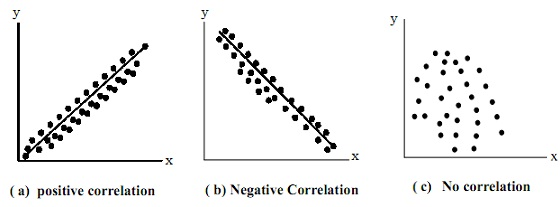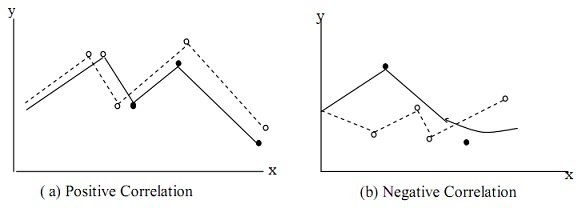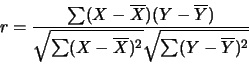#### Statistical Treatment of Data, Chemistry tutorial

Introduction:

In the earlier period, great chemist had faced different challenges, which comprise the necessity to analyze a reasonable large number of samples in so many monitoring effort in such a way to make sure representative coverage, choice of suitable method out of many acknowledged appropriate analytical methods, the problem of variations in reported values from various methodologies employed in analyzing the similar samples, coping with various measured values reported by the similar operation in replicate and un thinkable interrelationship which could exist among the data generated. Such problems and most of the others had forced scientist to just collecting 'base line' data for referral use, against which the future can be evaluated.

Though, it is now being realized that, by using the statistical analytical tools different problems could be solved. Statistics allows analytical chemist to accept conclusions which encompass high probability of being correct and to refuse conclusions that are doubtful. Therefore statistical treatment of data assists in ascertaining the importance and integrity of values reported.

Definitions of various Statistical Terms:

Significant figures:

It can be stated as the minimum number of digit needed to express a particular value in a scientific manner having a measured precision.

This theory is very significant in conveying the real meaning and status of each digit. Though most of the individual are not correctly schooled in the use of significant figures and therefore make figures in experimental reports appear confused. The digit zero (0) can be an important part of a measurement based on where it takes place.

Generally, zeros are noteworthy if:

1) They take place in the middle of a number.

2) They take place at the end of a number on the right-hand side of a decimal point.

It will be noted that the number of significant figures in a measurement is independent of the placement of decimal point.

Illustration: The significant zeros are underlined. 704, 0.0704, 0.704, 7040, 0.7040.

Ambiguity occurs whenever a figure such as 92500 is written in respect of the significant figures. Though it could be written in any of the given manners:

9.25 x 104          - 3 significant figures

9.250 x 104         - 4 significant figures

9.2500 x 104       - 5 significant figures

It will be noted that the first uncertain figure is the last significant figure.

=> Rounding Off:

The problem frequently encountered in significant figures is whenever an arithmetical operation occurs and when the answer is to be rounded off. The operation is addition or subtraction, multiplication or division.

The general rule is: Rounding must only be done on the final answer (that is, not in-between results) to avoid build-up of round-off errors.

Rule: Deduce all numbers with similar exponent and align all numbers with respect to the decimal point.

Round-off the answer according to the number of decimal places in the number having the fewest decimal places.

Illustration:

a)   14.344137

+ 17.347799

+ 44.313

76.504936 (not significant)

Whenever adding or subtracting numbers deduced in scientific notation, all numbers must first be converted or transformed to the similar exponent.

Illustration:

b) 1.373 x 105

+ 5.314 x 103

+ 0.798 x 106

Convert to the similar exponent:

1.373 x 105        1.373 x 105

5.314 x 103  →   0.05314 x 105

0.798 x 106        7.98 x 105

9.40614 x 105

Round off to smallest decimal points. Therefore, the final answer is 9.41 x 105.

=> Multiplication and Division:

The operation must be limited to the number digit contained in the number by the fewest significant figures.

Illustration:

a) 3.26 x 10-5

x 1.78

5.80 x 10-5

b) 4.3179 x 1012

x 3.6 x 10-19

1.6 x 10-6

c) 3 4. 6 0

x 2. 4 6 2 8 7

14. 0 5

It will be noted that the power of 10 has no influence on the number of figures that must be retained.

=> Rounding Off Rule:

It will be noted that rounding off must be done on the final answer, whenever the arithmetic operation should have been taken out.

The given rules validate rounding off operation.

1) If the digit following the last significant figure is more than 5, the number is rounded up to the subsequent higher digit.

2) If the number is less than 5, then the number is rounded to the present value of the last significant figure.

Illustration: 9.47 = 9.5 in two significant figures.

3) If the last digit is 5, then number is rounded off to the nearby even digit.

Illustration:  4.65 = 4.6 not 4.7 and 4.75 = 4.8

Ways of Expressing Precision:

Statistics has enabled scientist to accept or refuse conclusions on figures based on the degree of precision taken or attached by the numerical report.

Precision is stated as the degree of agreement between the replicate measurements of similar quantity. There are different tools which are uses in expressing the precision. These comprise: average deviation, variance, standard deviation and so on.

Average Deviation (A.D):

This is one of the procedures of showing dispersion or way of ascertaining the deviation from the central values. This is or else known as Mean Deviation. It assists further in measuring distribution which is based on all the items in a distribution.

Mean deviation or average deviation =

A.D        ∑|x - x‾|/n or ∑|dx‾|/n

Here,

dx = deviation from mean

n = number of observation

x = observation

x‾ = sample mean

Coefficient of mean deviation = Mean deviation/Mean

As with correctness, accuracy measurement such as average deviation can be represented as an absolute figure or as a relative figure (%, pph, ppt and so on).

Variance:

This is simply termed as mean square deviation. Variance is the significant measure in the quantitative analysis of data.  It assists in isolating the effect of different factors.  It as well assists in developing a few statistical theories.

Variance S2 = ∑(x - x‾)2/(n-1) or ∑ (dx‾)2/(n-1)

Here, x = arithmetic mean and n = number of observation

Standard Deviation (SD):

This is the most generally utilized absolute measure of dispersion. It evaluates how closely the data clustered about the mean.

Note:  The smaller the standard deviation the more closely the data is clustered about the mean that is, homogeneity is noticed when standard deviation is small.

Thus, standard deviation evaluates the spread in a set of observation. Standard deviation (S) is simply the square root of the variance.

S = √[∑(x - x‾)2/(n-1)] or √[∑(dx)2/(n-1)]

Coefficient of Variation:

Standard deviation is the absolute measure of dispersion. This is deduced in terms of unit in which the original data is collected. For illustration the standard deviation of length of fish is different from the standard deviation of weight of fish.  To enable comparison of the two, there is the requirement for conversion into relative measure. This relative measure of dispersion is termed as coefficient of variation.

C.V = (S/x‾ x 100)

Here S = Standard deviation and x‾ = Mean

Standard Deviation of Mean:

This is otherwise termed as standard error of mean (SEM).

SEM = S/√N

Here, S = Standard deviation and N = number of observation.

It will be noted that when the sample given throughout the measurement of dispersion is less than 10, we use (n - 1) however if it is more than 10, we make use of 'n'.

Student's t Test:

This is a statistical tool most frequently utilized to compare the mean values from experimental procedure. It as well assists in expressing confidence interval. This is the range in which the true value might fall in a given probability. The limit of this range is termed as confidence limit.  The possibility that the true value falls in the range is termed as the probability or confidence level, generally represented as a percentage.

A statistical t-value is computed (tcal) and compared by the tabulated t-value (ttab). If the  computed  t-value  surpasses  the  tabulated  t-value,  then  there  is  a  noteworthy difference between the results of the two methods at that confidence level. If it doesn't exceed the tabulated t-value, then we can calculate that there is no significant difference between the methods.

There are three modes by which t-test can be employed.

T-test when a standard or true value is known:

+t = (x‾ - µ) √(N/S)

Here,

x = mean value

µ = true value

N = number of observations

S = standard deviation

T-test if comparing replicate measurements:

+t = (x1‾ - x2‾)/Sp √[(n1n2)/(n1 + n2)]

Sp = √[(xi - x1‾)2 + (x2i - x2‾)2 + ...... (xki - xk)2/(n1 + n2 - k)]

Here,

x1, x2 ...xn, are the mean values of each of the set

SP = Pooled standard deviation

xi1, xi2... xik = Individual value in each set

K = sets of analyses

T-test if comparing the individual difference:

This case applies when we use two different methods to make single measurement on several different samples. No measurement has been duplicated.

t = (d‾/Sd)√n

Sd = √(di - d‾)2/(n-1)

d1 = Individual difference between the two methods for each samples with regards to sign

d‾ = Mean of all the individual difference.

F-test:

This is the test designed to investigate whether there is a significant difference between the two method based on their standard deviation commonly. This is defined in terms of variance.

F1 = S12/S22

Here, S12 > S22. If the computed 'F' value surpasses tabulated 'F' value at a given confidence level, there is a significant difference among the variance.

Correlation:

This is a statistical tool by the help of which the relationship between the two variables is studied. However, Correlation studies as well assist to show degree of any relationship (that is, quantitatively) between the two sets of variables.

With this knowledge, one can calculate if the existence of trend in one variable will influence the other. There are three main ways through which correlation is taken out.

• Scatter diagram method
• Graphic method
• Coefficient of correlation

1) Scatter Diagram Method:

This  is  the  simplest  process  for  ascertaining  correlation  between  the two  variables  via plotting the values on a chart termed as scatter diagram.

In plotting this diagram, note that the 'X' variable is the independent variable whereas on y-axis you plot the dependable variable.

Illustration: The height of plant is on x-axis as the number of flower is on y-axis.  The given types + scatter graph are generally obtained.Fig: Scatter diagram patterns of Correlation

2) Graphic method:

This is a mutual arithmetical graph in which the graphs of two variables in question are plotted and are related to one other. The graphs would either exhibit negative or positive correlation.Fig: Graphical Pattern of Correlation

3) Coefficient of Correlation:

This is if the degree of relationship can be established by computing a coefficient which for all time provides a quantitative measure of the degree of closeness among the two variables. This hypothesize is the basis for ranking numerical measure of degree of correlation. One of such numerical evaluated is Pearson Correlation coefficiency, 'r'.Here,

x = the independent variable

y = dependent variables

r = correlation coefficient

x and y are the mean values of the independent and dependent variables correspondingly

Though, for simplification purposes, the 'r' can be rewritten as:

The 'r' computed would exhibit the degree of the interrelationship going as pointed in table illustrated below.

Table: Interpretation of Degree of Correlation

 Degree of Correlation Positive Negative Perfect correlation +1 -1 Very high correlation + 0.9 or more - 0.9 or more Sufficient correlation + 0.75 to 0.9 - 0.75 to - 0.9 Moderate correlation + 0.6 to 0.75 - 0.6 to - 0.75 Possible correlation + 0.3 to 0.6 - 0.3 to - 0.6 Possibly No correlation Less than 0.3 Less than - 0.3 Absent of correlation 0 0

Tutorsglobe: A way to secure high grade in your curriculum (Online Tutoring)